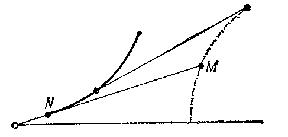# Curve of pursuit

A curve representing a solution of the "pursuit" problem, which is posed as follows: Let a point $M$ be moved uniformly along a given curve. The trajectory has to be found of the uniform movement of a point $N$, such that the tangent drawn towards the trajectory at any moment of the movement would pass through the position of $M$ corresponding to that moment in time.Figure: c027400a

In a plane, the system of equations which the curve of pursuit must satisfy takes the form

$$\eta-y=\frac{dy}{dx}(\xi-x),\quad F(\xi,\eta)=0,$$

where $dy/dx$ is the slope of the curve of pursuit, and $F(\xi,\eta)=0$ is the equation of the given curve.

The "pursuit" problem was posed by Leonardo da Vinci and solved by P. Bouguer (1732). For a generalization of it see the last Chapter of .